# 小白学 Python 数据分析（21）：pyecharts 好玩的图表（系列终篇）

2019/04/10 10:10## 引言

PS：顺便说一下， pyecharts 的文档质量非常好，所有的图表都有示例，对于刚接触的新手来讲十分友好，啥也不会的前提下，把 Demo CV 出来就能直接运行，学习代码，从 CV 开始。

## 3D 柱状图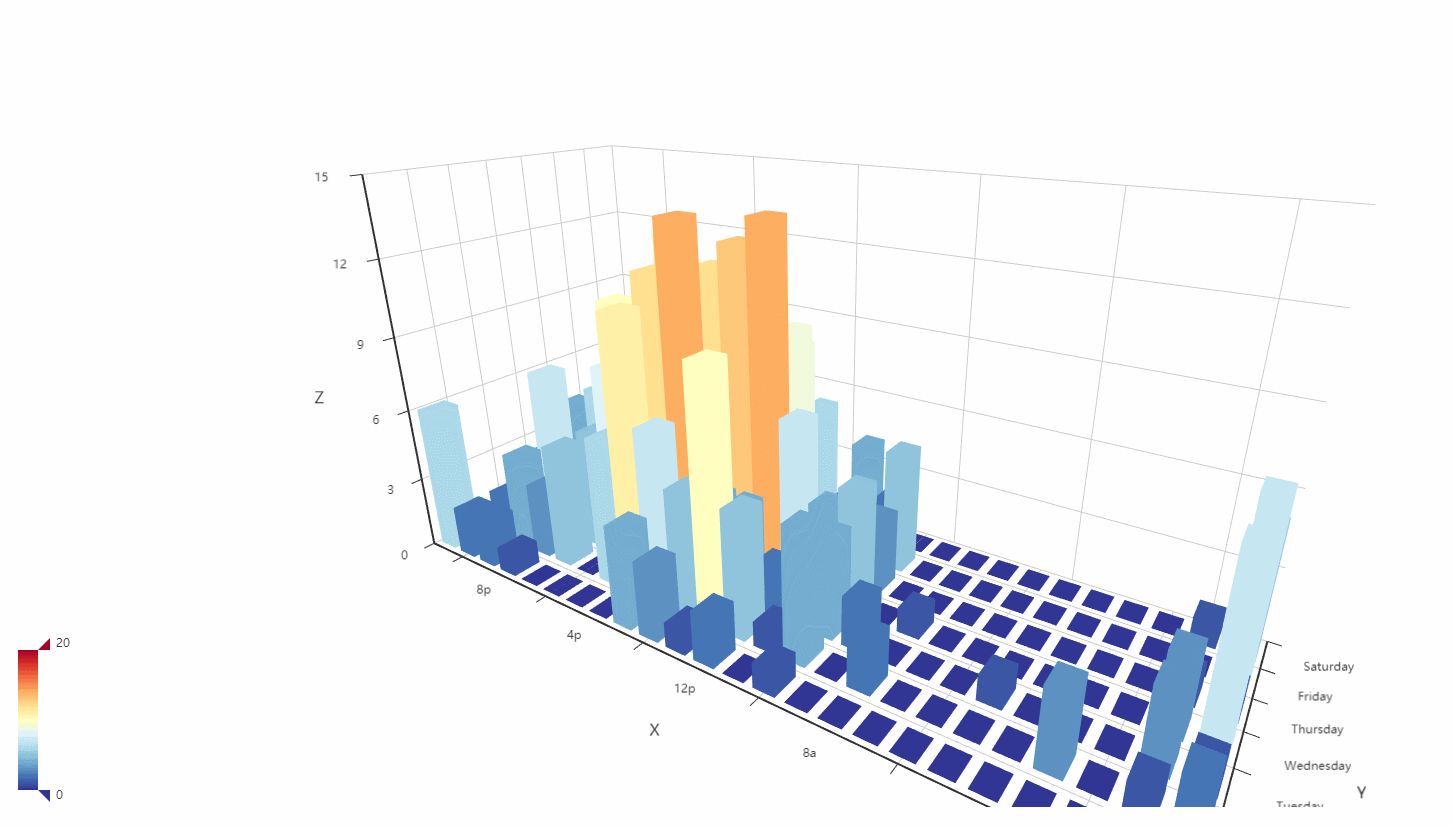import pyecharts.options as opts
from pyecharts.charts import Bar3D

# 省略部分数据代码

data = [[d, d, d] for d in data]

(
Bar3D(init_opts=opts.InitOpts(width="1600px", height="800px"))
series_name="",
data=data,
xaxis3d_opts=opts.Axis3DOpts(type_="category", data=hours),
yaxis3d_opts=opts.Axis3DOpts(type_="category", data=days),
zaxis3d_opts=opts.Axis3DOpts(type_="value"),
)
.set_global_opts(
visualmap_opts=opts.VisualMapOpts(
max_=20,
range_color=[
"#313695",
"#4575b4",
"#abd9e9",
"#e0f3f8",
"#ffffbf",
"#fee090",
"#fdae61",
"#f46d43",
"#d73027",
"#a50026",
],
)
)
.render("bar3d_punch_card.html")
)


## 日历图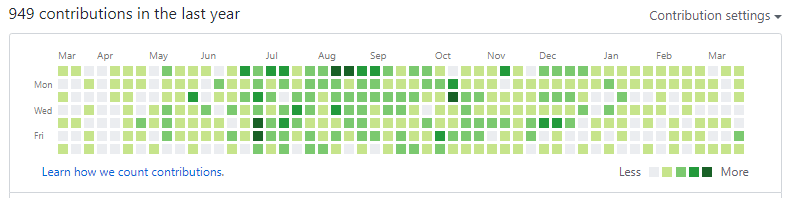import datetime
import random

from pyecharts import options as opts
from pyecharts.charts import Calendar

begin = datetime.date(2019, 1, 1)
end = datetime.date(2019, 12, 31)
data = [
[str(begin + datetime.timedelta(days=i)), random.randint(1, 20)]
for i in range((end - begin).days + 1)
]

c = (
Calendar()
.set_global_opts(
title_opts=opts.TitleOpts(title="Calendar-2019 Github 提交情况"),
visualmap_opts=opts.VisualMapOpts(
max_=20,
min_=1,
orient="horizontal",
is_piecewise=True,
pos_top="230px",
pos_left="100px",
),
)
.render("calendar_base.html")
)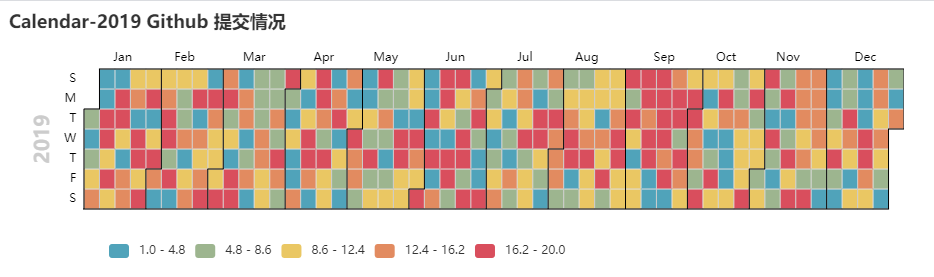## 仪表盘

from pyecharts import options as opts
from pyecharts.charts import Gauge

c = (
Gauge()
.set_global_opts(title_opts=opts.TitleOpts(title="Gauge-基本示例"))
.render("gauge_base.html")
)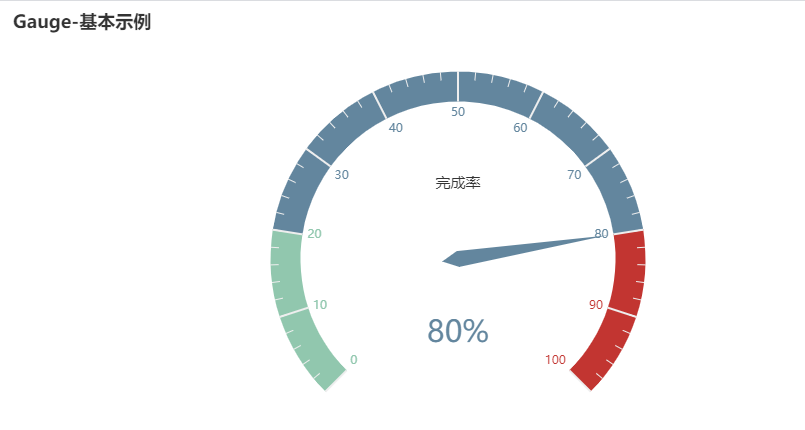## 关系图

from pyecharts import options as opts
from pyecharts.charts import Graph

nodes = [
{"name": "肖恩", "symbolSize": 10},
{"name": "海棠朵朵", "symbolSize": 20},
{"name": "长公主", "symbolSize": 30},
{"name": "陈萍萍", "symbolSize": 40},
{"name": "范闲", "symbolSize": 50},
{"name": "林婉儿", "symbolSize": 40},
{"name": "庆帝", "symbolSize": 30},
{"name": "范若若", "symbolSize": 20},
{"name": "司理理", "symbolSize": 10}
]
for i in nodes:
for j in nodes:
c = (
Graph()
.set_global_opts(title_opts=opts.TitleOpts(title="庆余年人物关系图"))
.render("graph_base.html")
)## 3D 折线图

import math

from pyecharts import options as opts
from pyecharts.charts import Line3D
from pyecharts.faker import Faker

data = []
for t in range(0, 25000):
_t = t / 1000
x = (1 + 0.25 * math.cos(75 * _t)) * math.cos(_t)
y = (1 + 0.25 * math.cos(75 * _t)) * math.sin(_t)
z = _t + 2.0 * math.sin(75 * _t)
data.append([x, y, z])
c = (
Line3D()
"",
data,
xaxis3d_opts=opts.Axis3DOpts(Faker.clock, type_="value"),
yaxis3d_opts=opts.Axis3DOpts(Faker.week_en, type_="value"),
grid3d_opts=opts.Grid3DOpts(
width=100, depth=100, rotate_speed=150, is_rotate=True
),
)
.set_global_opts(
visualmap_opts=opts.VisualMapOpts(
max_=30, min_=0, range_color=Faker.visual_color
),
title_opts=opts.TitleOpts(title="Line3D-旋转的弹簧"),
)
.render("line3d_autorotate.html")
)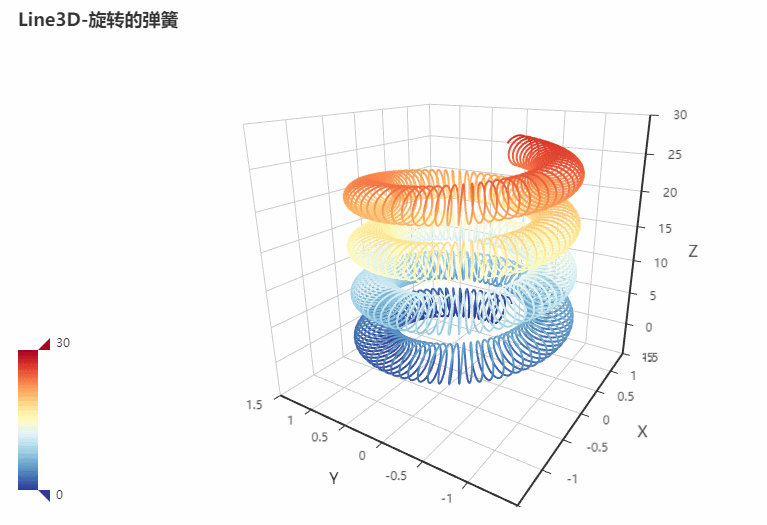## 水球图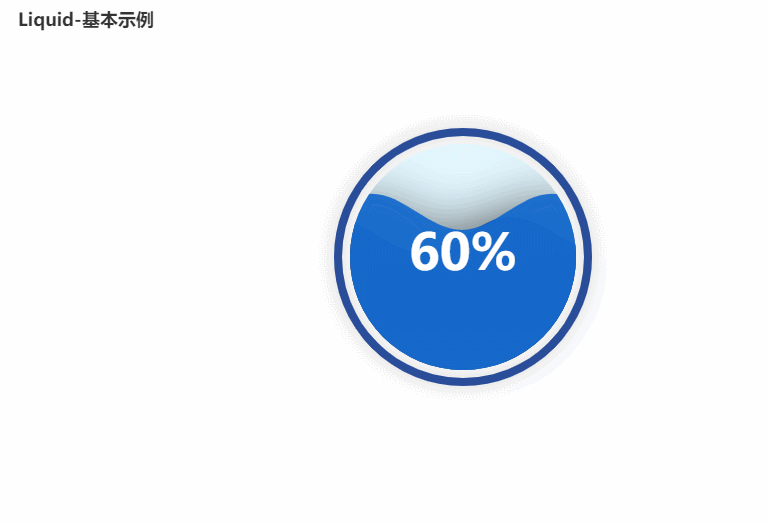from pyecharts import options as opts
from pyecharts.charts import Liquid

c = (
Liquid()
.set_global_opts(title_opts=opts.TitleOpts(title="Liquid-基本示例"))
.render("liquid_base.html")
)


## 百度地图

pyecharts 还支持百度地图上的一些操作，这对于需要使用地图做图的同学来讲不得不说是一个福音，比如下面的全国主要城市空气质量图：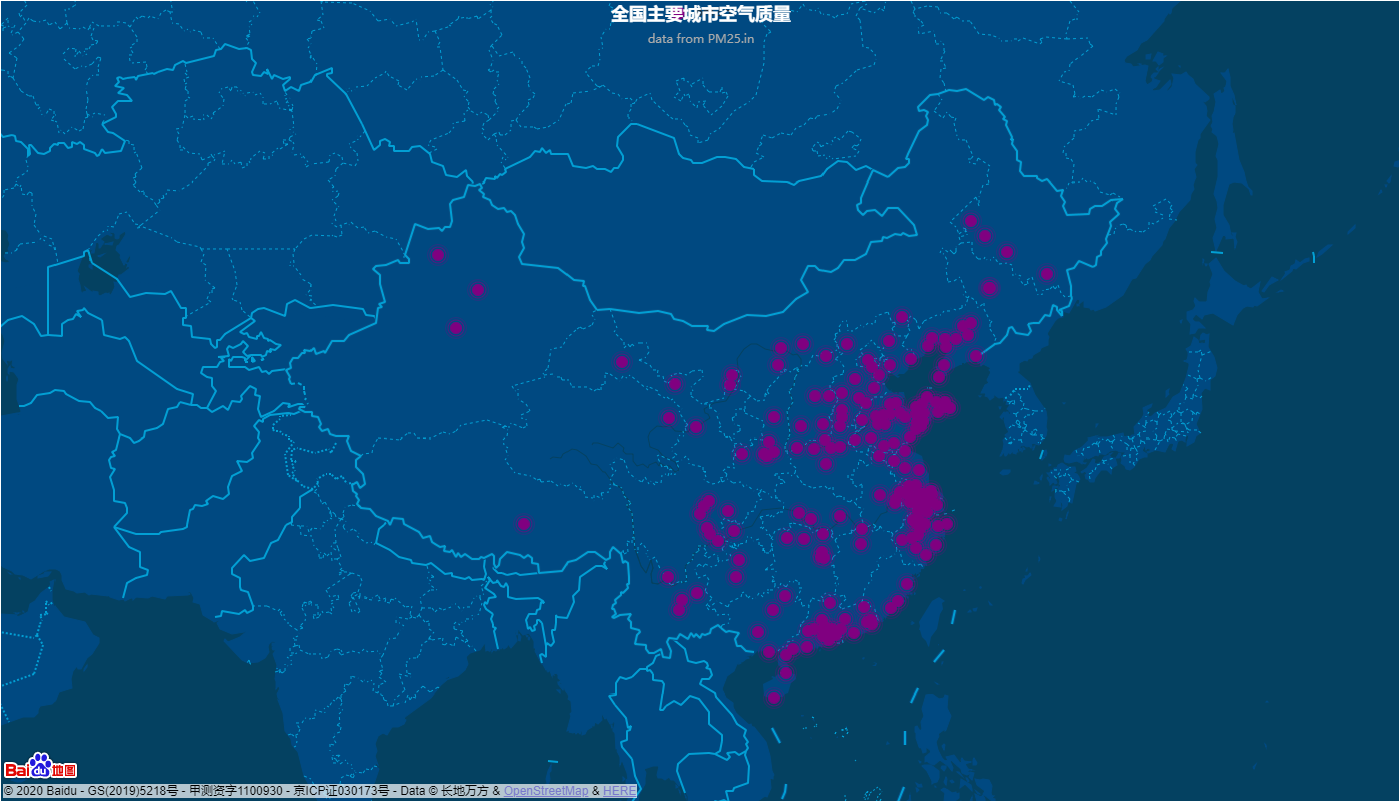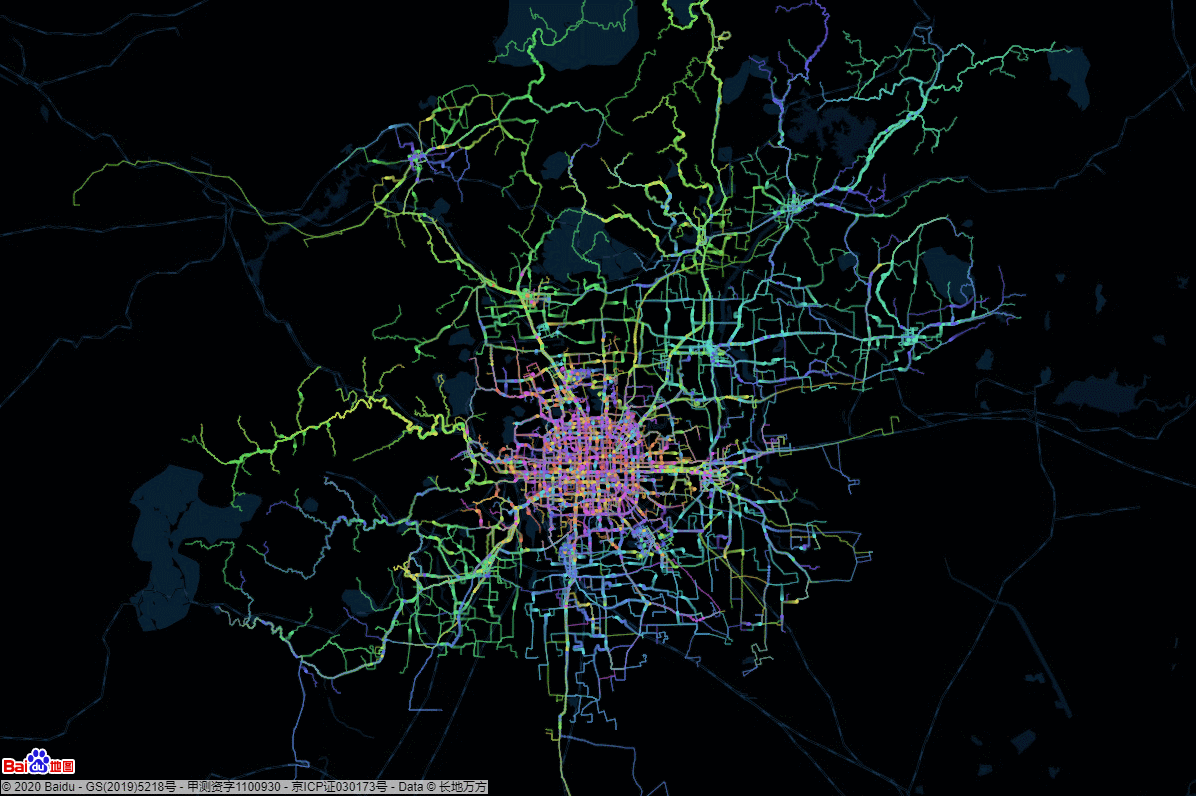## 多 BB 两句## 参考

0
1 收藏

### 作者的其它热门文章0 评论
1 收藏
0